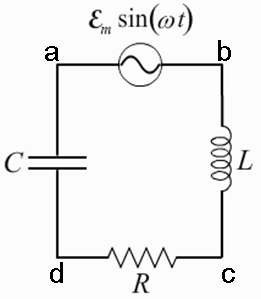# Problem: A circuit is constructed with an AC generator, a resistor, capacitor and inductor as shown. The generator voltage varies in time as ε = Va - Vb = εmsin(ωt), where εm = 120 V and ω = 737 radians/second. The values for the remaining circuit components are: R = 83 Ω, L = 112.5 mH, and C = 11.6μF.Given that the impedance of the circuit is Z = 89.7Ω, what is Imax, the magnitude of the maximum value of the current in the circuit?

###### FREE Expert Solution

The maximum value of the current is expressed as:

imax = εm/Z

96% (442 ratings)###### Problem Details

A circuit is constructed with an AC generator, a resistor, capacitor and inductor as shown. The generator voltage varies in time as ε = Va - Vb = εmsin(ωt), where εm = 120 V and ω = 737 radians/second. The values for the remaining circuit components are: R = 83 Ω, L = 112.5 mH, and C = 11.6μF.Given that the impedance of the circuit is Z = 89.7Ω, what is Imax, the magnitude of the maximum value of the current in the circuit?